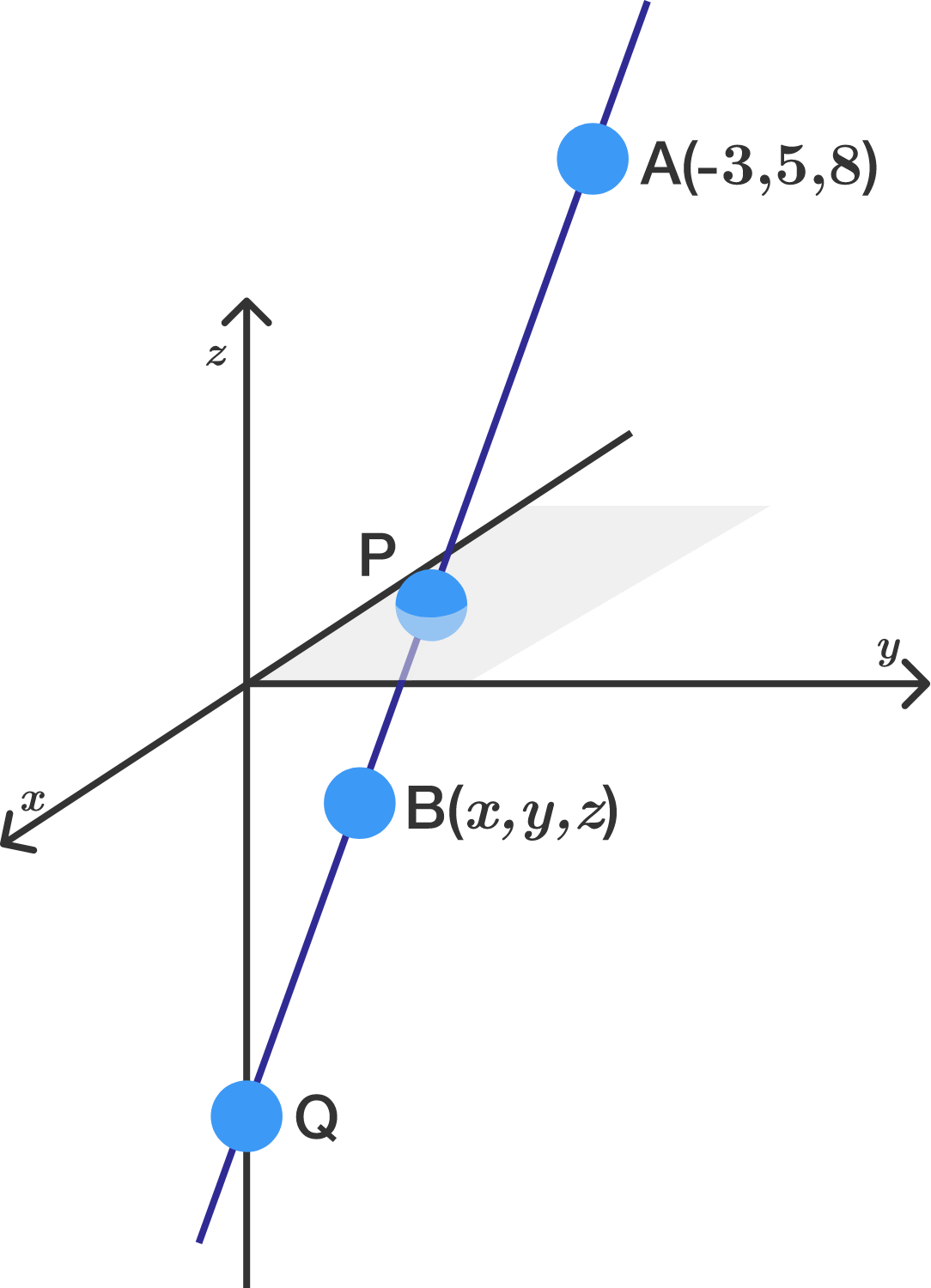# 3D points challenge

Geometry Level 2

The points $A$, $B$, $P$, and $Q$ all lie on one line, with $A=(-3, 5, 8).$

Point $P$ lies in the $xy$-plane between $A$ and $B$ such that the distance from $A$ to $P$ is twice the distance from $P$ to $B$. Furthermore, $Q$ sits on the $z$-axis such that the distance from $A$ to $Q$ is twice the distance from $Q$ to $B$.

If $B=(x,y,z),$ what is the value of $x+y+z?$×